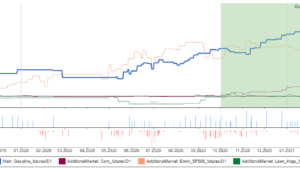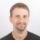# Better results with equity control?

Equity control functionality in Quant Analyzer allows you to simulate and test various methods of using equity curve to control trading logic.

What does it mean exactly?
The strategy can look at its own performance in the past (its equity curve so far) and use it to determine how it should trade in the future.
The premise is simple – can we improve the strategy future returns by analysing its past equity?

As an input we need a strategy (results) so we can try to improve its performance. In this post we’ll use the Test_EURUSD_strategy sample strategy with the equity curve displayed below.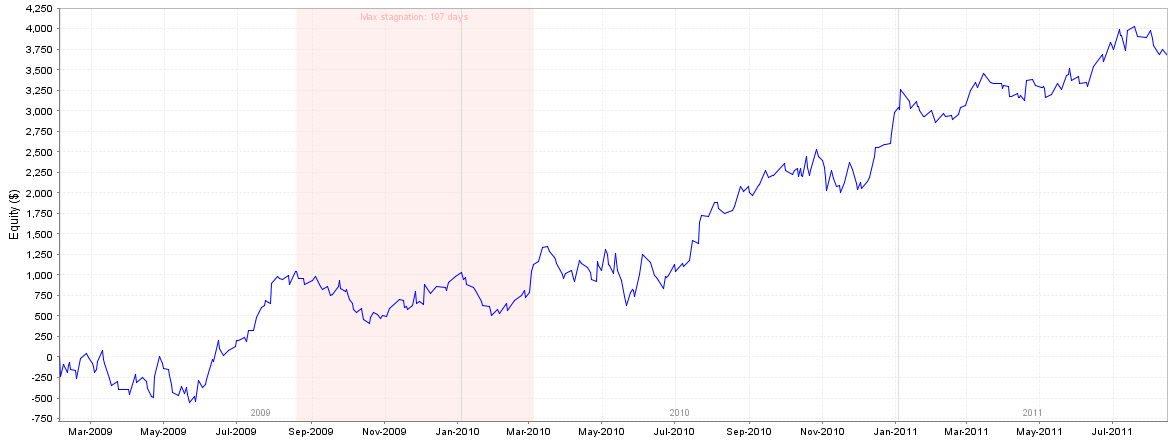When improving strategy performance the default approach usually taken is to try add one or more market filters, improve strategy entries or exits, reduce number of losing trades and increase number of winning trades.

But another interesting option that is the focus of this article is managing the equity curve of the system. In other words, we examine the past strategy performance and use this information to determine if we should take the next trade or not.

Example of Equity Curve management – simple approach using moving average
The idea is simple – add 20 period moving average to the equity curve generated by the strategy. Then employ the rules:

• If current equity curve is above its moving average and a new trade should be open then take the trade
• If current equity curve is below its moving average, then don’t take the trade for real, only take a paper trade and update the strategy equity curve

## Simulating equity control in Quant Analyzer

Quant Analyzer lets us easily simulate this in its Equity Control tab. Just choose the Equity Control type you want to use, set its parameters and click on Apply.
In our example we’ll use Moving Average with period 20.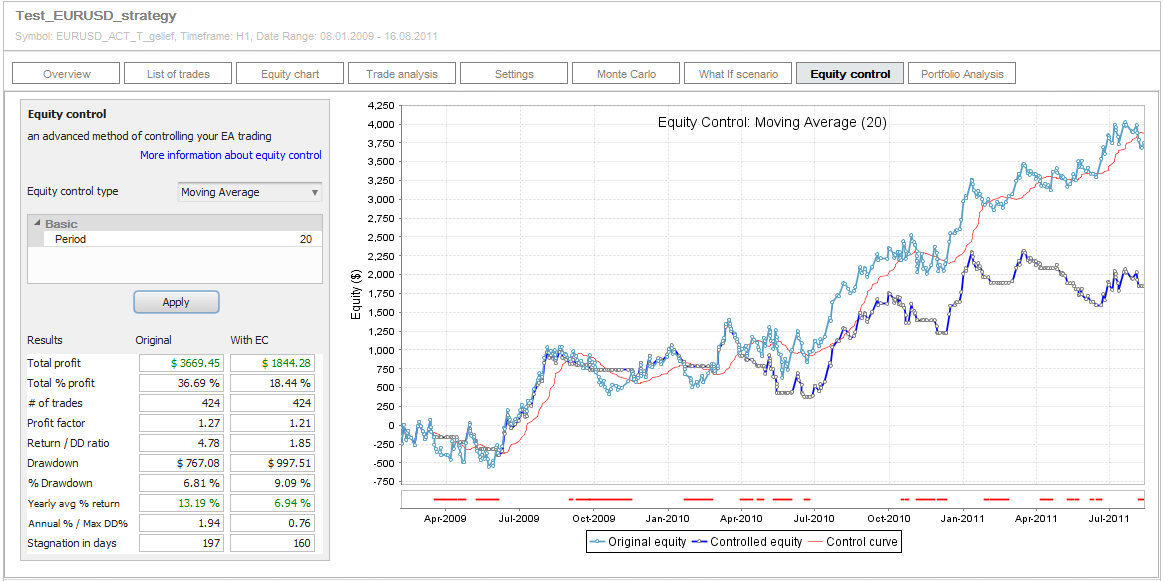After clicking on Apply we’ll get two curves – the original equity curve without any changes and the “Controlled” equity curve where we used these rules in trading.
You can also see the red control line – in our case 20 period moving average of original equity that decides if the trade is taken or not.

Special equity control chart
Please note that the equity curves on the chart are displayed in a special way – every point is a new trade and the equity level at that point is actual account balance at the trade open.
This enables us to control the trades using equity curve – we can decide if to take the trade according to the account balance when opening the trade – just like in reality.

If there are multiple trades opened at exactly the same time, they are all “grouped” to one trade point.

The red thick line on the very bottom of the chart clearly shows periods when the trades were not taken or changed by equity control.

By the look on the chart above we can immediately see that employing equity control this way doesn’t improve the strategy results, in fact it makes it worse. Let’s look at the details and zoom the chart to the first few months.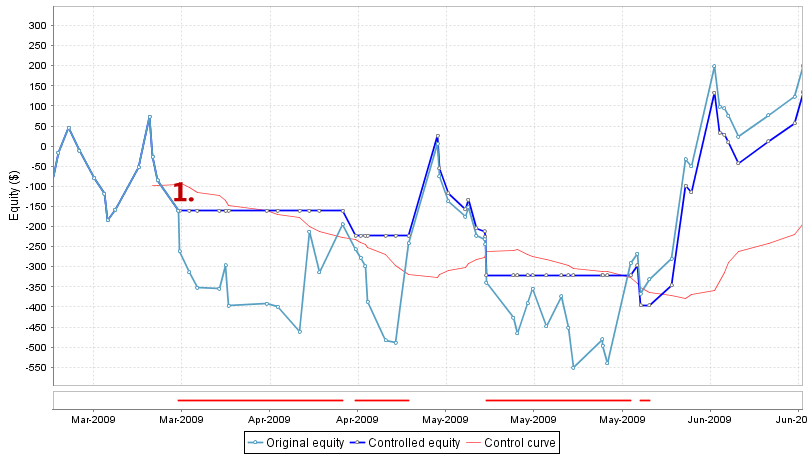Both original and controlled equity lines are the same until the point 1. From that day the equities differ:

• The original equity curve shows that the strategy continues trading and makes a further loss
• The controlled strategy doesn’t take the next few trades because the current equity at the trade open is below its moving average (red line). So the strategy passes the trades and prevents us to go into deeper loss until the original equity climbs up above its moving average.

So on this zoomed sample we can see that equity control works – it prevented strategy from going into bigger drawdown by missing the trades when original equity curve was below its moving average.

So why is the overall result of controlled strategy worse than the original one?
Let’s look at another section of the chart starting at around March 2010: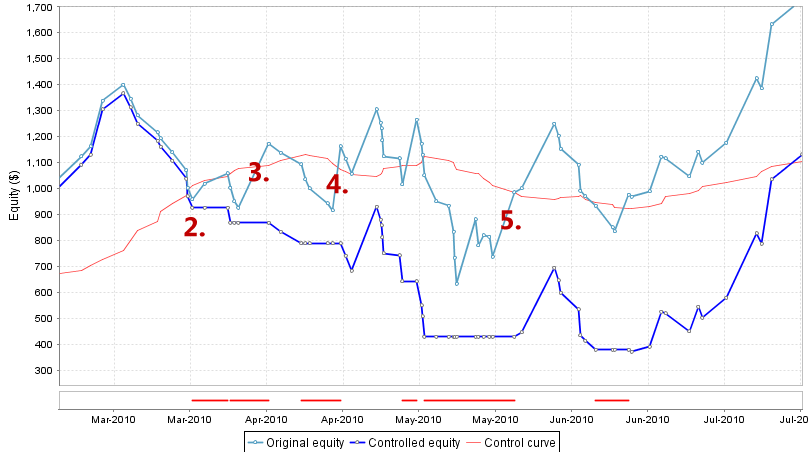Again, both original and controlled equity lines are roughly the same until the point 2.. From then on the “Controlled” strategy starts losing its performance compared to original strategy.

Why is that?
Our rules specify that the strategy doesn’t trade when its equity is below its moving average. This means it might skip some losing trades preventing further loss, but it can also skips some winning trades that helped original strategy to recover from previous losses.

In our case it misses very profitable trades 3., 4. and 5. because at the time when these trades should have been opened the original equity curve was below its moving average.
Controlled strategy will take the next trade only after the original equity climbs back above its moving average.

## Conclusions of this example

Our equity control simulation shows that this kind of equity control doesn’t improve anything. In fact it almost always decreases the profit and also increase drawdown if not used right.

But what about if we’ll try something else?
Instead of missing a trade when equity is below average we can double its size. The rules will be simple again:

• If current equity curve is above its moving average and a new trade should be open then take the trade with its original size
• If current equity curve is below its moving average and a new trade should be open then open the trade with double size

In this case we are speculating that after few losses there should come a profitable trade and we’d use double size to get double profit from it.

Extending Quant Analyzer
To create new custom equity control function we’ll use extendability of the newest version of Quant Analyzer.
Quant Analyzer v3 now allows you to programm your own equity control function and test your own ideas. In this example we’ll use special equity control function that you can download below.

Simply download this file, place it into {Quant Analyzer}/extend/code/EquityControl folder and restart the program.

You should see the new option Moving Avg Add Contracts added in the equity control types selection. You can leave the parameters unchanged, the parameter Coef means the coefficient by which we’ll increase the trade size if equity is below its moving average. It uses formula:

new trade size = coefficient * original size;

By setting it to 2 we’ll double the trade size.
Click on Apply and we can check the simulation results.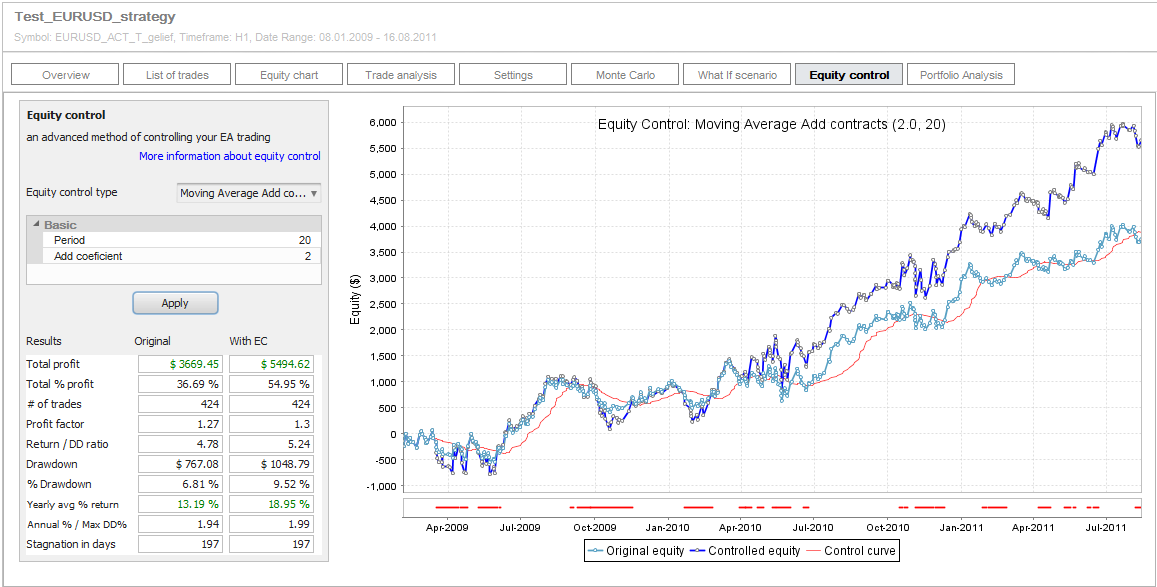We can see that this time the net profit of strategy increased significantly, but it also increased the drawdown a little.
We can take it as an example that there is a room to influence strategy performance significantly by managing the trades using its past equity chart.

## Final thoughts

The approach and examples we tried in this article shows just one of the many possibilities of using equity control with your strategy.
Quant Analyzer comes with 2 other equity control functions and it allows you to create your own ones to test your own ideas.

What to watch for
There are some disadvantages of equity control that we should be aware of:

• It is very difficult to implement in EA – it is easy to simulate this kind of control in Quant Analyzer, but it is another task to implement it in your EA. In fact I don’t know any EA that would use similar approach of managing trades using its equity.
• It depends on the original strategy logic – it will not work the same on every strategy, it depends on things like Risk-Reward ratio (size of SL vs PT), the “look” and steepness of the equity curve, number of consecutive winning and losing trades, and so on.
• It sometimes doesn’t work well in “chops” – when the equity goes sideways the controlled strategy might lose more than the normal one, because of its tendency of missing profitable trades because equity is below its average.

Overall, it is a technique worth considering, and Quant Analyzer allows you to simulate the potential results before you’ll start the task of implementing it in your strategy.

Inline Feedbackscomparison blocks

### Creating consecutive blocks in a Strategy Quant XclonexJune 28, 2021

In StrategyQuant X 131, we have added two new comparison blocks. In this article we will discuss them in more detail. Comparison blocks are snippets that we can use in the Algo wizard or  Builder and are used to be able to compare two values. ( Left and Right )### How to build and backtest strategies in MultiCharts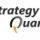Tomas VanekJune 15, 2021

Performing backtest of the strategy in the trading platform is a necessary step before going live. Generally, all trading platforms are performing the same tasks which are providing the environment …